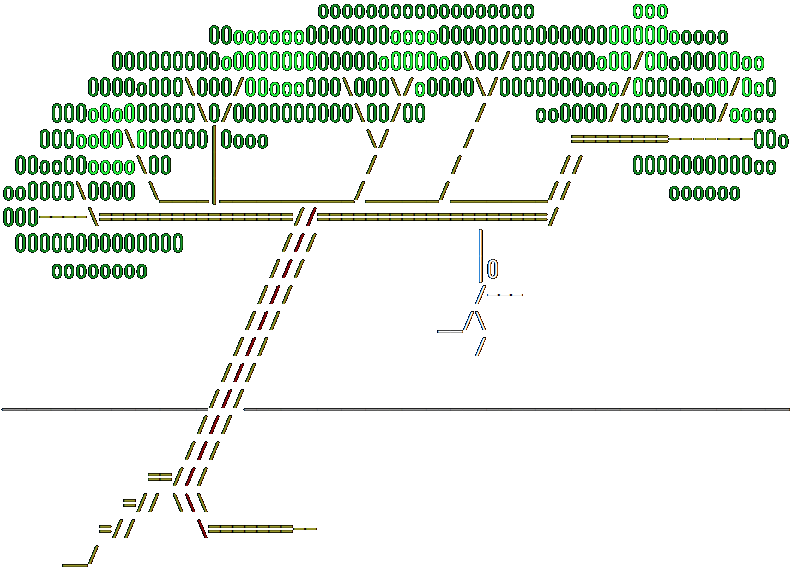# Realer than Real: Exact Real ConstantsModern computers are fundamentally binary devices, whereas we humans tend to think in decimal terms. For many intents and purposes it is possible to place a decimal façade over the binary logic, but inconsistencies show through at the edges. Nowhere is this more true than for binary floating point arithmetic, and I would argue that unless you want to have a bad time, you should think in binary terms anytime you mess with floats or doubles, known in Hemlock as `r32` or `real`/`r64`. In this post I focus on the nuances of numerical constants embedded in source code, i.e. the constant values programmers write in their code.

Consider this constant taken from some 1980s-era numerical code: `3.343_057_558_358_812_810_5e4`. That’s 19 digits past the decimal, yet the computer is only capable of 15-17 decimal digits of accuracy. Here the author provided extra digits with the hope that rounding during conversion to binary would be correct. This plagued programmers for decades, and eventually the problem was mitigated  via some very clever math (with some subtle practical implications I won’t get into) that determines the minimum extra precision required to facilitate correct rounding. But in my opinion the problem only ever existed due to path dependence, i.e. we collectively made the wrong decision long ago to keep pretending computers perform decimal floating point math. Hemlock steps back from this unfortunate status quo, eschews precisely rounded decimal floating point constants in favor of bit-precise binary floating point constants. Furthermore, the compiler warns the programmer if the binary constant cannot be exactly represented!

### Realer

Here is where the Hemlock compiler’s `Realer` module comes in. When the compiler scans a `real` literal, it has to be prepared for unreasonably precise constants, as well as exact constants with many, many digits. For example, `0x0.0000_0000_0000_0000_0000_0000_0000_0001` can be precisely represented, and things can get a lot crazier; I won’t deface this post with `0x1p-1074` in binary radix point format. The `Realer` module incrementally computes the mantissa as a `nat` value and the exponent as a `zint` value. These are arbitrary-precision values, so the only practical limit is available memory. Once the mantissa and exponent are known, it’s a relatively simple matter to compute how many binary digits would be required to precisely represent the value (subnormals complicate things slightly). If the value can’t be precisely represented, the compiler complains.

The decimal-formatted constant shown earlier rounds to `0x1.052d_26b2_e45e_4p15`, which requires 50 bits of mantissa precision. If you extend that to, say, `0x1.052d_26b2_e45e_4fp15`, 56 bits of mantissa precision are required. `Realer.t` can of course handle that, but `real` maxes out at 52 bits, so the conversion is lossy.

1. Wichura, M.J. “The percentage points of the normal distribution,” Applied Statistics 37(3):477-484, 1988.

2. David M. Gay, “Correctly Rounded Binary-Decimal and Decimal-Binary Conversions,” AT&T Bell Labs, Numerical Analysis Manuscript 90-10, November 30, 1990.